Grant Maloy Smith

Tuesday, December 1, 2020 · 0 min read

# What is Power Analysis and Electrical Power Measurement With Power Analyzer

In this article, we will look into what power analysis is and the tools that are used to perform it. In this article you will:

• See what electrical power really is

• Learn why we need power analysis and how it is calculated

• Understand how power analysis is measured by a power analyzer

Are you ready to get started? Let’s go!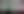## What is power analysis?

Power is the rate of doing work, i.e., the energy consumed per unit of time. The power of an electrical system is the multiplication of the voltage with the current, integrated over and then divided through periodic time. The periodic time (equals the frequency) must be known in order to calculate the power of an electrical system. “Power analysis” is simply the method by which power is tested and studied, typically using a power analyzer.

## What is a power analyzer?

A power analyzer is an instrument that measures and quantifies the rate of power flow in electrical systems. Power flow is expressed in Joules/second (J/s) or kilowatt-per-hour (kW/h). Electrical power is the rate per unit of time that electrical energy is transferred in an electrical system between two points.SIRIUS XHS Power Analyzer with 4x high-voltage and 3x low-voltage amplifiers for current transducer connection

## What is electrical power?

You can look at an electric circuit, but you cannot see if the voltage is present or if a current is flowing. You must not reach out your hand to find out, because this is extremely dangerous and possibly even lethal. Therefore we must use the correct instrument to measure electricity.

So how can we visualize electricity moving through a circuit? Well, we can see the water moving, so let’s use it as an analogy to explain how electrical circuits work. It is a well-known fact that if the water is to flow out of a pipe, the water needs to have a force or “pressure” pushing it, either from gravity or a mechanical pump.

In our analogy:

• Voltage is the pressure that forces the water through the pipe. The higher the pressure the faster the water will flow. This is measured in volts (V).

• The current is the available volume for the water to flow in. The larger the volume the more water can flow. This is measured in amperes (A).

• Resistance is volume reduction inside the pipe that restricts the flow of water. This is measured in ohms (R or Ω).

If the current is moving only in one direction, it is very much like water flowing through a pipe or hose. This is DC (direct current) in our analogy. However, if the current moves back and forth, then it is analogous to AC (alternating current)

AC power is what we use to transport electricity over long distances, from the power plant to our homes and businesses, for example.

DC power is used for modern electronics as well as batteries.

The office computer you might be reading this on, for example, plugs into AC power, but it has a type transformer inside known as a switched-mode power supply (SMPS) that converts AC to DC power and converts the DC voltage to the desired level. If you’re using a notebook computer, the SMPS is likely located in the external “brick” that connects the AC outlet on the wall and the DC power system inside the notebook. If you’re reading this on a phone or tablet, it is also a DC device that uses an external SMPS to charge its internal battery.

## Quantifying electrical power

In physics, electric power is the rate of doing work. It is equivalent to the amount of energy consumed per unit of time. The unit for power is Joule per second (J/s), also known as Watt (W).

### What is electrical power

Electrical power is the rate per unit of time that electrical energy is transferred in an electrical system between two points. The first law of thermodynamics states that energy cannot be created or destroyed. It can merely be converted from one type of energy to another, or be transferred

Since no ideal electrical system exists, there will always be some losses when there is a transfer of energy. The most common form of loss within an electrical system is heat. If a circuit is physically warm, that means that some of the energy that it is carrying is being converted into heat, and therefore cannot be used to do useful work.

This decreases the efficiency of the overall electrical system. It is not a coincidence that mechanical systems also generate heat - don’t put your hand on a lit incandescent bulb, or you will directly experience the energy conversion to heat. Electrical power is just an extension of the basic physics of power in general.

Conventionally electric power is expressed in kilowatts (kW).

## How do we calculate electrical power?

The amount of power in a circuit is calculated by multiplying the Voltage (V) with the Current (A) which yields Watt (W), using the following equation:

$P(t)=I(t)\cdot V(t)$

This basic equation can be transformed using ohm’s law, which states that the current flowing through a linear resistance is directly proportional to the voltage and inversely proportional to the resistance of an electrical circuit at a constant temperature. Ohm’s law can be written in several ways:

$V=I\cdot R$
$P=V\cdot I$
$P=I^2\cdot R$
$P=\frac{V^2}{R}$

But ohm’s law is only true for direct current (DC), where the flow of voltage and current is constant.

But with alternating current (AC), the ohm’s law equations will yield the power at only one instant in time. So we need a different method of measuring AC.

We need an equation that accurately describes electrical power if we want to analyze its characteristics. Luckily, there is such an equation:

$P=\frac{1}{T}\int^T_{t=0}u(t)\cdot i(t)\,dt$

Where:

• P is power in Watt (W)

• i is current in Ampere (A)

• u is the voltage in Volt (V)

• T is the periodic time in seconds (s)

Let’s visualize this equation on a graph:Power calculation equation visualized on a cartesian plane showing the voltage and current, and the resulting power curve after integration.

Looking at the curvature of the waveforms in the visualization we can see that the power in an AC system is not just voltage multiplied by current as in a DC system. It is defined by the time average of the instantaneous power over one cycle. This means that we must know the frequency in order to calculate the power of an electrical system.

## Understanding power measurement

Principally there are three types of power in alternating current (AC) electrical systems to be measured. These are:

1. Active power (P)

2. Reactive power (Q)

3. Apparent power (S)

To illustrate the relationship between them there is a handy tool that we can use, known as the power triangle, based on the Pythagorean Theorem:Power triangle, illustrating the relationship between active, reactive, and apparent power, including the angle phi and the power factor, also known as cosine phi (cos phi)

Let’s look deeper into these terms and what they really mean:

### What is active power (P)

Active power (P) also known as “real power” or “active power” is the useful power that is used within the AC circuit.

### What is reactive power (Q)

Reactive power (Q) is not used but is transported between the source such as a power station and the load, it is mainly used to transport the active power through the electrical system.

### What is apparent power (S)

Apparent power (S) is the vector sum of active and reactive power in an AC power system.

### What is the power factor (PF)

The power factor (PF) is the ratio between active and apparent power and can take on values between 1 and -1.

The Power factor is an indication of the amount of active power that is present in the transmission line compared to the apparent power that combines both the active and reactive power. In other words, it is the factor by which the useful power in the transmission line is less than the maximum power theoretically possible. Reductions in the theoretically ideal power factor are caused by the voltage and current being out of phase.

The Power factor is often  denoted as “cos phi,” “cosine phi” or “cos 𝜑.”

Reactive power can be positive or negative, indicated by the positive or negative sign of the angle phi (𝜑). This tells us if the current is leading the voltage, or if it is lagging behind the voltage in the transmission line.

When the reactive power value is positive it is lagging, indicating an inductive load that is consuming reactive power.

When the reactive power value is negative it is leading, indicating a capacitive load that is delivering reactive power.

Pure ohmic loads, like traditional incandescent light bulbs, have a power factor very close to 1. This means that voltage and current are in phase, so there is very little reactive power present in the transmission line.

With positive power factors, the closer they get to zero, the larger the phase difference between voltage and current, and the more reactive power is present in the transmission line. This is similar to the negative power factor, just in the opposite direction: at  PF = -1  the phase difference between voltage and current is 180°.

## Power vs. energy - what’s the difference?

The terms “electric energy” and “electric power” are not interchangeable, because they are not the same thing. Using our water analogy from earlier, it’s easy to illustrate this difference:

Power means capacity while energy represents delivery over time

Power is essentially the flow rate of water in the hose, based on its pressure and volume. Electric power is measured in watts (W), kilowatts (kW), and megawatts (MW).

Energy is the amount of water that comes through the hose over a period of time. That’s why your electric bill will be given in kilowatt-hours (kWh).

## Why do we measure power?

Measuring voltage and current is only the initial step to analyzing an electrical system, and can easily be done with any power analyzer or power meter on the market.

But in order to manage something successfully, one needs as much information as possible. This is exactly what a power analyzer is designed to do. Power analyzers make it easy for the user to perform complex analyses of any electrical system with only a few operations.

As electricity and power become more and more important, it is critical that it can be measured and managed to the highest standards possible to ensure that the supply continues and that the equipment that operates using it is reliable, safe, and efficient. From energy production itself to the transmission phase that brings it to our homes and businesses, power analyzers are critical to making accurate and comprehensive measurements.

Measuring power to the highest possible level of precision is important for various reasons:

• For R&D to increase the performance of products and services

• To increase energy efficiency

• Reducing cost and time consumption

• Compliance with national and international standards

• Ensuring the safety of products and operators

## What do power analyzers do?

Power analyzers conduct a wide range of tests and measurements on electrical components, circuits, and systems. Some of the most common analyses that are done include:

Load Flow Analysis is used to establish the components of a power system which include voltage magnitude, current magnitude, the phase angle phi of the system, active power, reactive power, apparent power, and the power factor in a steady-state operation.

Additionally, for non-linear loads, distortion reactive power as well as harmonic reactive power need to be measured and analyzed. In theory, voltage and current have a perfect 50 Hz sine wave in Europe (and 60 Hz mostly in North and South America). This is the case if there are only pure ohmic linear loads connected to the grid (e.g. incandescent light bulbs, electrical heaters, AC electromotors, etc.).

The power triangle that was shown previously only holds true for ohmic loads, but currently, there are more and more non-linear loads as well as non-linear production units connected to the grid. This has introduced a new dimension into the power triangle namely distortion and harmonic reactive power.

Let’s take a look at the new power triangle:The new power triangle illustrates the relationship between active, reactive, and apparent power, including the new dimension of distortion and harmonic reactive power

In the example below, the line voltage feeds AC power into the system, and the switching rectifier converts it to the DC power that the LED requires. Take a look at the schematic diagram of the measurement setup:Schematic diagram of a LED testing power measurement set-up with both the AC and the DC voltage and current waveforms measured with the power module from Dewesoft

Currently, there are more and more nonlinear loads (ballast units, rectifiers, inverters, personal computers, etc.) connected to the grid, as well as nonlinear generation units (wind, solar, and other forms of energy generation). Therefore, the waveforms of voltage and current are distorted and not ideal sinusoidal waveforms. Therefore, harmonic analysis is necessary to determine the effects that these nonlinear loads have on the current and voltage in an electrical system.

Short circuit analysis is done to provide information on all the possible operating scenarios of the electrical system and to ascertain the capacity of individual components in the system to interfere with or withstand the magnitude of the current in the circuit.

Coordination analysis is used to support the development of overcurrent protection. It takes into consideration the characteristics of the protection device, including its sizing and settings, in order to establish the ideal operating range.

## Dewesoft power analyzers

The Dewesoft Power Analyzers are not only the smallest power analyzers in the world, but it’s also the most powerful ones. The flexible hardware platform combined with powerful software features gives unique testing possibilities for any kind of electrical measurement. The Dewesoft power analyzer can calculate more than 100 power parameters, such as P, Q, S, PF, cos phi, and many others.

It also offers several features of other instruments:

• Raw data recording capabilities

• Oscilloscope

• FFT analysis

• Harmonics

• etc.

All these calculations can be done online in real-time, in post-processing, or both.The Dewesoft Power analyzer combines multiple instruments and functionalities in a single device - power analyzer, FFT analyzer, RAW data recorder, oscilloscope, harmonics analyzer, temperature recorder, vibration recorder, and many more.

The Dewesoft R8 power analyzers can be equipped with up to 64 high-speed analog inputs (up to 1 MS/s @ 16-bit and 2 MHz bandwidth per channel) for voltage and current measurement in a single box.The Dewesoft R8DB power analyzer can be configured with 64 channels, selectable to suit the measurement application for an all-in-one measurement device

The inputs are fully isolated both on the sensor side (channel to the ground), as well as channel-to-channel, and even isolated sensor excitation. Real galvanic isolation means less noiseground loop avoidance, and superior signal quality

High voltages can be directly measured by our high voltage inputs with 1600 V DC/CAT II 1000 V/CAT III 600 V protection. Current can be measured with high-accuracy current sensors, like zero-flux current transducers, AC/DC current clamps, Rogowsky coils, and shunts.Dewesoft provides a broad range of current transducers and currents sensors for any current measurement range and accuracy

And while it is mainly a power analyzer, it can also measure various additional signal types, including accelerometers, strain gage, force, and load sensors, thermocouples, RTDs, counters and encoders, GPS, CAN BUS, XCP, FlexRay, and even video. All channels are synchronized with each other.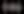What Is a Strain Gauge Sensor and How Does It Work?Strain and pressure sensors are widely used in many static and dynamic measurements. Learn the best practices and theory behind strain gauge measurement.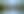How to Measure Weight With Load Cell SensorsSee how load cell sensors work. Learn how weight measurements are made in science and industry. Understand how you can incorporate them into your testing.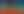What Is a Thermocouple Sensor and How Does It Work?In this article, you will learn what is a thermocouple, which are the basic types available, and how the temperature is measured with these sensors today.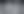How To Measure Temperature with RTD Sensors [PT100, PT200, PT1000, ...]Learn about RTD sensors (Resistance Thermometer Detectors), types of RTD sensors, how they work, and how to measure temperature with Dewesoft DAQ systems.

## The SIRIUS XHS - the next-generation power analyzer

The SIRIUS XHS power analyzer is the latest version of the SIRIUS product line. It is a high-speed data acquisition system capable of recording at 15 MS/s/ch and up to 5 MHz bandwidth on all analog inputs.The new SIRIUS XHS shown with four HV amplifiers and four LV amplifiers

It features an all-new HybridADC technology that is capable of high-bandwidth transient recording and very high-dynamic, alias-free data acquisition. Alias-free filtering allows the acquisition of signals with up to 160 dB dynamic range. High channel-to-channel and channel-to-ground isolation prevents damage to the systems from excessive voltage and avoids ground loops.

The new HybridADC Technology inside SIRIUS XHS power analyzer

In most power applications, the SIRIUS XHS is configured with four high-voltage (HV) and four low-voltage (LV) amplifiers:

• SIRIUS XHS HV: Highly isolated CAT II 1000V high voltage analog input. This amplifier can measure ranges from 20 V to 2000 V peak directly, with 5 MHz bandwidth and an accuracy of 0.03%. This amplifier is ideal for the direct connection of high voltage signals. The connectors of this amplifier are always insulated safety banana jacks (red/black).

• SIRIUS XHS LV: Highly isolated low voltage analog input. This amplifier can measure ranges from 0.05 V to 100 V, with a 5 MHz bandwidth, an accuracy of 0.03%, and excitation for selected sensors (requires DSUB9 connector for sensor excitation). This amplifier is ideal for the direct connection of low voltage signals and current transducers. The connectors of this amplifier are available in  DSUB9 or BNC. Note that the DSUB9 connector also offers sensor excitation as well as TEDS for smart sensor setup.

## Power analyzer with built-in FFT analyzer

Conventional power analyzers use zero-point detection to determine the periodic time. This means that they evaluate when the voltage or current crosses that x-axis and use that value to calculate the periodic time.

Dewesoft on the other hand uses a special FFT (Fast Fourier Transform) algorithm to determine the periodic time (frequency).

Based on this predetermined period time, an FFT analysis of voltage and current can be done for a definable number of periods (typically 10, if the system’s base frequency is 50 Hz), and at a selectable sample rate. The FFT analysis yields an amplitude for the voltage, current, and cos phi for each harmonic.The Dewesoft power module has a built-in FFT analyzer in addition to the other visual display types

## Multi-phase power analyzers

In the DewesoftX power module, there are several predefined system configurations available to choose from. The most common ones are:

• Direct current,

• 1-phase

• 2-phase - is used, e.g. with special types of motors

• 3-phase star

• 3-phase delta

• Aron and V configuration is basically star and delta configurations but measuring only two currents instead of three. This is normally done as a space-saving or cost-reduction measure.

Special configurations such as 6-, 7-, 9- or 12-phase motor measurements can be done with multiple single-phase or 3-phase systems and add up the power values in the Math library. This means that power can be measured at multiple points completely synchronously.

In the math library, the power modules can be further refined, for example, the efficiency can be calculated automatically. This is also very helpful when measuring multi-phase motors (6 to 12 phases).The Dewesoft power module can be configured for 1, 2, and 3-phase systems. These can be combined to create 6, 7. 9 or even 12-phase systems

Engineers can simply select one or more of the systems that they are measuring from this list:

• 1-Phase

• 2-Phase

• 3-Phase star

• 3-Phase delta

• 3-phase Aron

• 3-phase V

• 3-phase 2-meters

In addition, a wide range of other choices is available, including the line frequency, output units, frequency source (the channel to be evaluated to determine the exact frequency), phase, and more.

Due to the modular design of Dewesoft measurement devices, the user is never limited to only measuring power values. Dewesoft DAQ systems can connect to virtually every sensor in the world, which means that the engineer can also measure temperature, force, vibration, sound, GPS, video, speed, RPM, torque, etc.The Dewesoft power analyzer wiring schematic for testing inverter and electric motors

Engineers performing tests on electric or hybrid vehicles may also want to measure the speed of the car, the battery temperature, CAN bus data, and GPS position, and even plot its exact location on a test track.

Instead of using two, three, or even more different measuring instruments, Dewesoft offers all measurements to be recorded simultaneously within a single instrument. This brings several key advantages:

• No need to merge the data together manually after the measurement.

• Data is completely synchronized down to a single sample.

• All the data can be viewed on one screen and written into one data file.

• Configuring and using only one DAQ system and software saves a lot of test preparation time.

Dewesoft power analyzer explained at the Battery Show expo